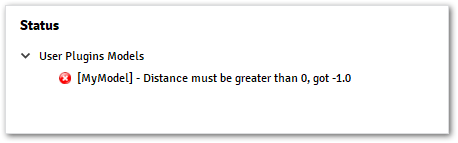# Application Hooks#

The application hooks allow plugins to add custom models or custom checks in the application. To add an application hook it is necessary to implement the given hook in a python file that is already available on your plugin project folder.

As an example, if the `alfasim-sdk new` command was created with the name `myplugin`, the necessary file to be customized will be located on: myplugin ‣ src ‣ python ‣ myplugin.py

## Plugin Model#

alfasim_get_data_model_type()#

This hook allows the creation of models in ALFAsim, models can:

• Customize items on ALFAsim application, by adding new components over the Tree.

• Hold input data information to be accessed from the solver.

• Validate input data or configuration made on ALFAsim, to ensure that the plugin has all configuration necessary to be run successfully.

This hook needs to return a class decorated with one of the following options:

The image below shows the locations where a custom model can be inserted implementing the hook.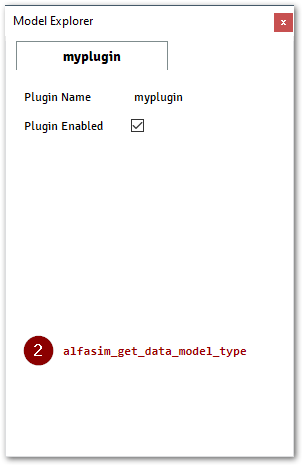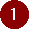Location to where the models `container_model()` or `data_model()` will be placed.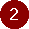Location to where the inputs fields will be placed.

Example 1: The following example shows how to create a new model.

```import alfasim_sdk
from alfasim_sdk import data_model
from alfasim_sdk import Quantity

@data_model(icon="", caption="My Plugin")
class MyModel:
distance = Quantity(value=1, unit="m", caption="Distance")

@alfasim_sdk.hookimpl
def alfasim_get_data_model_type():
return [MyModel]
```

The image below shows the output of example 1 when executing on ALFAsim.Example 2: This second example shows how to create a new container model.

Notice that when using the `container_model()` you only need to inform the container class to the `alfasim_get_data_model_type()` hook

```import alfasim_sdk
from alfasim_sdk import data_model, container_model
from alfasim_sdk import Quantity, String

@data_model(icon="", caption="My Child")
class ChildModel:
distance = Quantity(value=1, unit="m", caption="Distance")

@container_model(icon='', caption='My Container', model=ChildModel)
class MyModelContainer:
my_string = String(value='Initial Value', caption='My String')

@alfasim_sdk.hookimpl
def alfasim_get_data_model_type():
return [MyModelContainer]
```

The image below shows the output of example 2 when executing on ALFAsim.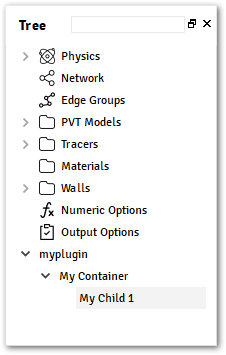Example 3: This third example demonstrates that it’s possible to create multiple models within the plugin

```import alfasim_sdk
from alfasim_sdk import data_model, container_model
from alfasim_sdk import Quantity, String

@data_model(icon="", caption="My Model")
class MyModel:
distance = Quantity(value=1, unit="m", caption="Distance")

@data_model(icon="", caption="My Child")
class ChildModel:
distance = Quantity(value=1, unit="m", caption="Distance")

@container_model(icon='', caption='My Container', model=ChildModel)
class MyModelContainer:
my_string = String(value='Initial Value', caption='My String')

@alfasim_sdk.hookimpl
def alfasim_get_data_model_type():
return [MyModelContainer, MyModel]
```

The image below shows the output of example 3 when executing on ALFAsim.## Status Monitor#

alfasim_get_status(ctx)#

Allows plugins to execute custom checks on ALFAsim. These checks can be used to guarantee the consistency of the data or compatibility with some configuration made on ALFAsim.

The status monitor accepts two types of message:

`ErrorMessage`:

Signalize the application to block the simulation until the error is fixed.

`WarningMessage`:

Signalize the application that the user needs to fix this problem, but does not need to block the simulation.

When no errors are detected, an empty list must be returned.

The `alfasim_get_status()` will be called for:

• Each time a model from the plugin is modified.

• Each time a `Physics options` from ALFAsim are modified.
Ex.: Hydrodynamic model changed

The `ctx` parameter is provided to retrieve information about the current state of the application and the current value of the models implemented by the user.

Check out the full documentation of `Context` for more details.

The following example shows how to display an ErrorMessage when a `Quantity` field does not have the desired value.

```import alfasim_sdk
from alfasim_sdk import data_model
from alfasim_sdk import Quantity
from alfasim_sdk import ErrorMessage

# Define MyModel used in this plugin
@data_model(icon="", caption="My Plugin Model")
class MyModel:
distance = Quantity(
value=1, unit="m", caption="Distance"
)

@alfasim_sdk.hookimpl
def alfasim_get_data_model_type():
return [MyModel]

# Add status monitor in the plugin
@alfasim_sdk.hookimpl
def alfasim_get_status(ctx):
results = []
distance = ctx.GetModel("MyModel").distance.value
if distance < 0:
message = f"Distance must be greater than 0, got {distance}"

results.append(
ErrorMessage(
model_name="MyModel",
message=message,
)
)
return results
```

For the status monitor above, the application will show the following message when the distance is less than 0: